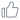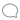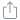# 浅谈Tensorflow加载Vgg预训练模型的几个注意事项

```Value passed to parameter 'input' has DataType uint8 not in list of allowed values: float16, bfloat16, float32, float64。
```

```image_path = "data/test.jpg" # 本地的测试图片

# 一定要tf.float()，否则会报错
image_decoded = tf.image.decode_jpeg(image_raw)

# 扩展图片的维度，从三维变成四维，符合Vgg19的输入接口
image_expand_dim = tf.expand_dims(image_decoded, 0)

# 定义Vgg19模型
vgg19 = VGG19(data_path)
net = vgg19.feed_forward(image_expand_dim, 'vgg19')
print(net)
```

```image_path = "data/test.jpg" # 本地的测试图片

# 一定要tf.float()，否则会报错
image_decoded = tf.to_float(tf.image.decode_jpeg(image_raw))

# 扩展图片的维度，从三维变成四维，符合Vgg19的输入接口
image_expand_dim = tf.expand_dims(image_decoded, 0)

# 定义Vgg19模型
vgg19 = VGG19(data_path)
net = vgg19.feed_forward(image_expand_dim, 'vgg19')
print(net)
```

```image_path = "data/3.jpg"
image_unit8 = tf.image.decode_jpeg(image_raw)
image_float = tf.to_float(image_unit8)

with tf.Session() as sess:
image_unit8_, image_float_ = sess.run([image_unit8, image_float])

print("image_unit8_", image_unit8_)
print("image_float_ ", image_float_ )
```

``` image_unit8_
[180, 192, 204],
[183, 195, 207],
[186, 198, 210],
...,
[191, 205, 218],
[191, 205, 218],
[190, 204, 217]],

image_float_
[180., 192., 204.],
[183., 195., 207.],
[186., 198., 210.],
...,
[191., 205., 218.],
[191., 205., 218.],
[190., 204., 217.]],
```

```import tensorflow as tf
from matplotlib import pyplot as plt
image_path = "data/boat.jpg"

image_decoded = tf.image.decode_jpeg(image_raw)
image_decoded = tf.to_float(image_decoded)

with tf.Session() as sess:
image_decoded_ = sess.run(image_decoded)
plt.imshow(image_decoded_)
plt.show()
``````save_path = "data/boat_copy.jpg"
image_uint = tf.cast(image_decoded, tf.uint8)
with tf.Session() as sess:
with open(save_path, 'wb') as img:
image_saved = sess.run(tf.image.encode_jpeg(image_uint))
img.write(image_saved)
```20分享